# Improving the Performance of Solar Air Heater Using High Thermal Storage Materials

Improving the Performance of Solar Air Heater Using High Thermal Storage Materials

Abdulmunem R. AbdulmunemAkram H. Abed Hashim A. Hussien Pakharuddin Mohd Samin Hasimah Abdul Rahman

School of Mechanical Engineering, Faculty of Engineering, Universiti Teknologi Malaysia, 81310 UTM Skudai, Johor, Malaysia

Ural Federal University Named after the First Persident of Russia B.N.Yeltsin 19Mira str., Yekaterinburg 620002, Russia

Electromechanical Engineering Department, University of Technology, Baghdad 10066, Iraq

Center of Electrical Energy System, School of Electrical Engineering, Faculty of Engineering, Universiti Teknologi Malaysia, 81310 UTM Skudai, Johor, Malaysia

Corresponding Author Email:
Page:
389-394
|
DOI:
https://doi.org/10.18280/acsm.430605
14 September 2019
|
Accepted:
26 November 2019
|
Published:
23 December 2019
| Citation

OPEN ACCESS

Abstract:

The solar air heater is a widely used thermal application of renewable energy. Various thermal storage materials, either sensible or latent, could be adopted for solar air heater, so that the thermal energy can be saved at sunny hours for use after the sunset. Considering its latent heat of fusion, paraffin wax, a phase change materials (PCM) was selected to store latent thermal energy, applied on a forced convection solar air heater (airflow: 4.47 kg/s), and compared with a sensible thermal storage material under the meteorological conditions of Baghdad city. Three tests were conducted on February 24, 25 and 27, 2019, respectively. In the first test, no thermal storage material was used; in the second test, pure cement was adopted as the sensible thermal storage material (specific heat: 1.55 kJ/kg·K; thermal conductivity: 0.29 W/m·K); in the third test, paraffin wax was selected as the latent thermal storage material (solid phase: specific heat: 2.0kJ/kg·K; thermal conductivity: 0.24 W/m·K; liquid phase: specific heat: 2.15kJ/kg·K; thermal conductivity: 0.22 W/m·K). The results show that the latent thermal storage material of paraffin wax achieved better thermal performance than the sensible thermal storage material of pure cement; the solar air heater with paraffin wax had a 50% higher thermal storage efficiency and realized twice the heating period after 6:30pm than that with pure cement.

Keywords:

solar air heater, storage heat, phase change materials, thermal storage efficiency

1. Introduction

In the present decade, the world's depended on fossil fuels more than 77% of total energy, the fossil fuels have an effect on the environment by polluting, greenhouse gases released and contribute to global warming. While solar energy is clean and free, so recently the world to utilize it instead of conventional fuels (fossil). Store energy from the sun is important to reuse it at sunshine time. There are many different materials using to store thermal energy in many different applications such as industrial, chemical, agricultural, residential use, etc. Storage heat materials can be classified into sensible storage heat materials and latent storage heat materials. Sensible storage heat materials were used for decades, now a day the researchers tend to utilize latent heat of fusion for phase change materials to save energy. Paraffin is an organic material with many advantages such as thermal and chemical stability, corrosives resistance, nontoxic, high fusion heat and low vapor pressure .

There are different works used thermal storage materials (sensible, latent) in solar energy applications such as solar collectors. A solar air heater was designed and developed to save energy with Paraffin wax as phase change material by Enbie , the study was done with natural convection and indicated that the rising in useful efficiency was (50%) with using the paraffin wax. A prototype solar cooker was investigated by Sharma et al. , the solar collector based on an evacuated tube with PCM (phase change material) as thermal storage material. It was observed that noon cooking does not affect evening cooking, and evening cooking by using PCM as heat storage material was found faster than noon cooking. Mahmud et al.  gives indoor simulation study (theoretically) of using a paraffin wax with addition 5% of aluminum powder, the study was simulated cylindrical shape absorber solar air heater and shows that the decreasing in output air temperature through the discharge process was with air mass flow rate increases, the compound (paraffin wax & 5% aluminum powder) take longer solidification time to with lower airflow. Saravanakumar et al.  present solar air heater thermal performance using gravel, iron scraps as sensible storage heat materials. The experimental results show that solar air heater with storage heat materials is suitable for drying crops. Abdulmunem et al.  shows experimentally under meteorological conditions of Baghdad city the thermal performance of a forced convection flat plate solar air heater with sand, concrete and pure cement as sensible heat storage materials. The results give that pure cement was better sensible thermal performance than other (sand, concert) for store energy.

This work aimed to evaluate and compare the overall thermal performance of flat plate solar air heater, using thermal storage materials (pure cement as sensible) and (paraffin wax as latent), and to estimate the thermal storage efficiency of the used system.

2. Theoretical Concepts

2.1 Collector useful heat energy gain

The calculation of the energy aspect for flat plate solar air heater can be summarized as follows: [7, 8].

$Q_{u}=\dot{m}_{\dot{a} i r} \times C_{p a i r} \times \Delta T_{a}$$=\dot{m}_{\dot{a} i r} \times C_{p a i r} \times\left(T_{a i r o u t}-T_{a i r i n}\right) (1) \dot{m}_{\dot{a} i r}=\rho_{a i r} \times A_{d} \times V (2) \rho_{a i r}=\frac{P_{a t m}}{R \times T_{a i r}} (3) \eta_{\text {collector}}=\frac{Q_{u}}{G \times A_{c}} (4) where, Qu is the useful heat energy from the collector (W), \dot{{m}}_{{\dot{a}ir}} is the air mass flow rate (kg/s) Cpair is the specific heat of the air (J/kg. K), ra is the air density (kg/m3), Ad is the outlet duct cross sectional area of the flat plate solar air heater (m2), \mathrm{P}_{\mathrm{atm}} is the atmospheric pressure (N/m2), R is the gas constant (8.314 J / mol·K.), Ac is the collector area (m2), \eta_{\text {collector }} is the collector efficiency (%) and G is the irradiance (W/m2). 2.2 Collector heat energy losses To absorb thermal energy by storage heat materials, the heat energy losses from the collector playing an important role and effect on the thermal performance, these losses can be classified into: 1- Heat energy loss from the collector to ambient 2- Heat energy loss from the absorber plate to glass cover. 2.2.1 Heat energy loss from the collector to ambient Thermal loss from the collector to ambient can be found by John . Q_{\operatorname{loss}}=U_{L o s s} A_{c}\left(T_{\text {plate}}-T_{\text {air}}\right) (5) U_{L o s s}=U_{t}+U_{b}+U_{e} (6) U_{t}=\frac{1}{\frac{N_{g l a s s}}{\frac{C}{T_{p l a t e}}\left[\frac{T_{p l a t e}-T_{a i r}}{N_{g l a s s}+f}\right]^{0.33}+\frac{1}{h_{w}}}}+\frac{\sigma\left(T_{p l a t e}+T_{a i r}\right)\left(T_{p l a t e}^{2}+T_{a i r}^{2}\right)}{\frac{1}{\varepsilon_{{p l a t e+0.05 N}_{g l a s s}}\left(1-\varepsilon_{p l a t e}\right)}+\frac{2 N_{g l a s s}+f-1}{\varepsilon_{g l a s s}}-N_{g l a s s}} (7) where, \mathrm{Q}_{\text {loss }} is the collector energy losses(W), ULoss is the overall heat loss coefficient from the collector area (W/m2.k) and is the sum of heat loss coefficient from the top \left(U_{t}\right), bottom U_{b}, and edges U_{e} of the collector. Ac is the collector area (m2), Tplate and Tair is the plate and air temperature (oC), h_{w} is the convective heat transfer coefficient by the wind (W/m2.k), N_{g l a s s} is the number of glass cover, \varepsilon_{g l a s s} and \varepsilon_{p l a t e} is emittance of the glass cover (0.88) and absorber plate (0.15). 2.2.2 Heat energy loss from the absorber plate to glass cover Q_{\text {loss,p-g}}=A_{c}\left(h_{c, p-g}+h_{r, p-g}\right)\left(T_{\text {plate}}-T_{\text {air}}\right) (8) h_{c, p-g}=\frac{\left(\begin{array}{c}{1+1.446\left[1-\frac{1708}{R a * \cos (\theta)}\right]} \\ {*\left\{1-\frac{1708[\sin (1.8 \theta)]^{1.6}}{R a * \cos (\theta)}\right\}} \\ {+\left\{\left[\frac{R a * \cos (\theta)}{5830}\right]^{0.333}-1\right\}}\end{array}\right) * K_{a i r}}{-L_p-g} (9) \bar{T}^{3}=\frac{1}{4}\left(T_{\text {plate}}^{2}+T_{\text {glass}}^{2}\right)\left(T_{\text {plate}}+T_{\text {glass}}\right) (10) \eta_{\text {storage}}=\frac{\text { Useful heat energy gain }}{\text { Useful heat energy gain }+\text { Heat energy loss }}$$=\frac{Q_{u}}{Q_{u}+Q_{l o ss}}$   (11)

$\mathrm{Q}_{\mathrm{loss}, \mathrm{p}-\mathrm{g}}$ is the heat energy loss from the absorber plate to glass cover of the collector(W), hc,p-g is the convection heat transfer coefficient from the absorber plate to glass cover (W/m2.k). hr,p-g is the radiation heat transfer coefficient from the absorber plate to glass cover (W/m2.k). $\overline{\mathrm{T}}$ is the average temperature(oC). Kair is the air thermal conductivity (W/m.k).

2.2.3 The uncertainty analysis of the measured values

For the measured values in this work, it can analyze and estimate the uncertainties for these values using the following formula [10-16].

$\mathrm{U}=\sqrt{\left(^{\partial \mathrm{F}} / \partial \mathrm{v}_{1}^{*} \mathrm{u}_{1}\right)^{2}+\left(\partial \mathrm{F} / \partial \mathrm{v}_{2} * \mathrm{u}_{2}\right)^{2}+\cdots+\left(\partial \mathrm{F} / \partial \mathrm{v}_{\mathrm{n}} * \mathrm{u}_{\mathrm{n}}\right)^{2}}$  (12)

where, F is a given function of the independent variables v1, v2 to vn, with U the uncertainty in the result u1, u2 to un, represents the uncertainties of each independently measured variable as shown in Table 1.

Table 1. Uncertainties of the measured values

 Parameters Quantity Uncertainty (U) Radiation (G) 1.2% Temperature (T) 1% Voltage (V) 0.5% Current (I) 0.5%
3. Experimental Setup

3.1 System configuration

To show the effect of using thermal storage materials on flat plate solar collector performance, a new solar air heater system combines a collector and an energy storage unit that was designed and fabricated. The experimental system consists of a flat plate collector integrated with an energy storage container, air intake system, data acquisition instruments, and support frame. Energy storage container with dimensions 60 cm width, 100 cm length, and 4 cm depth, which made from a wood and all sides painted with R.T.V silicon to avoid drainage the latent heat storage material during the melting process.

The thermo physical properties of paraffin wax and pure cement are shown in Table 2. An aluminum absorber sheet with a thickness of 0.5 cm was placed directly above the energy storage container and painted with matte black to increase absorption solar radiation. Single transparent glass 0.4 cm thickness placed as a cover of flat plate solar collector with a 10 cm gap between glass and aluminum sheet. The bottom side of the collector is opened with dimension of 54 cm and 7.5 cm, while the top side is opened with 7.5 cm diameter connected to the centrifugal blower fan to make air forced circulation. To control the air mass flow rate, a voltage transformer was used. The experimental work carried out under the methodological condition of Baghdad, Iraq latitude 33.310o N, longitude 44.450o E. The experimental tests were done in three cases: the first case without thermal storage material at 24 February 2019, the second case with pure cement as sensible thermal storage material at 25 February 2019 and third case with paraffin wax as latent thermal storage material at 27 February 2019. Figure 1 and Figure 2 shows configuration and the schematic layout of the system, respectively.

## 1.jpg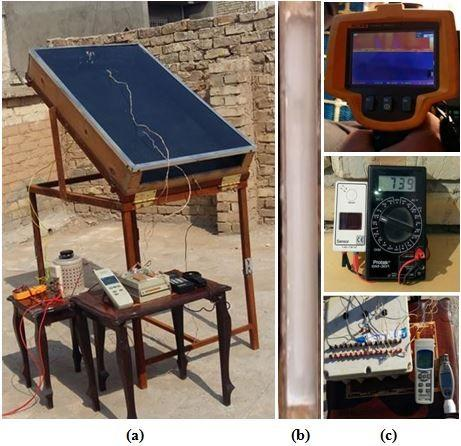Figure 1. System configuration: a) apparatus photo, b) collector container filled with paraffin wax, c) measurement instruments

Table 2. The thermo-physical properties of (paraffin wax) and pure cement

 Material Specific heat (kJ/kg.K) Thermal conductivity (W/m.K) Density (kg/m3) Melting temperature [°C] Latent heat of fusion [kJ/kg] Paraffin wax Solid (2.0) Liquid (2.15) Solid (0.24) Liquid (0.22) Solid (910) Liquid (790) 53.7 190 Cement-dry 1.55 0.29 1506 ----- -----

## 2.jpg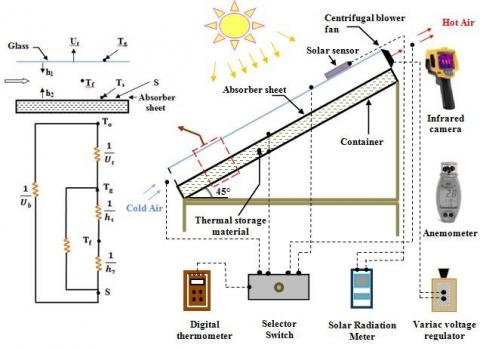Figure 2. Schematic layout

3.2 Experimental instrument's measurement

An anemometer vane probe-type Lutron AM-4206 with accuracy ±4% was used to measure air velocity. To measure the solar irradiation, the solar meter instrument type Protek / DM-301 was used with solar intensity range from 0 to 2000 W/m2 and accuracy ±0. 7%. The infrared camera type Fluke-Ti300 with accuracy ±2% was used in this work. Also, five calibrated thermocouples type K were used to measure the absorber surface, glass, inlet, and outlet air temperatures. All five thermocouples are connected to a manual selector switch, then to digital thermometer type BTM-4208SD with accuracy ±0. 01%.

4. Discussion of the Results

To investigate the aim of the present work, it must make a comparison between selective test materials, pure cement (sensible) and paraffin wax (latent) in the solar air heater, with the case of no storage heat materials. The tests were done on three sunny days 24, 25 and 27 February 2019, under the methodological condition of Baghdad city, and the recorded irradiation data are shown in Figure 3. Figure 4 gives thermocouples data of collector glass cover, absorber temperature compared with ambient air temperature for the first test day on 24 Feb. (Collector without storage heat materials), this curve indicates that the system works properly (heating of air) to begin on the next tests.

At 25, 27 Feb, a forced convection test with 4.47 kg/sec of airflow with the storage heat materials (pure cement, paraffin wax) respectively, Figures 5 and 6 shows the absorber temperature and outlet air temperature for two collectors compared with the first case (collector without storage heat), it seen that the absorber temperature of collector with pure cement at charging time for interval time  6:30 AM to 2:30 PM is less than the absorber temperature of the collector without storage heat materials because some of the heat undergoes to storage heat materials.

## 3.jpg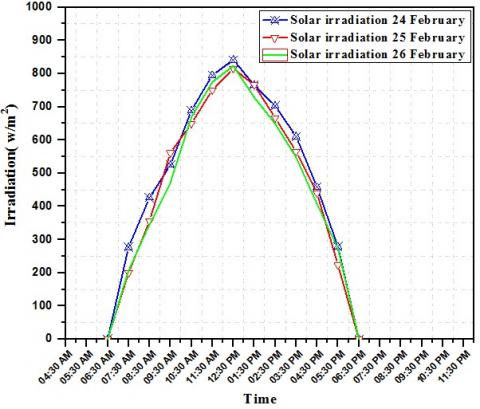Figure 3. Distribution of solar irradiation for three test days

## 4.jpg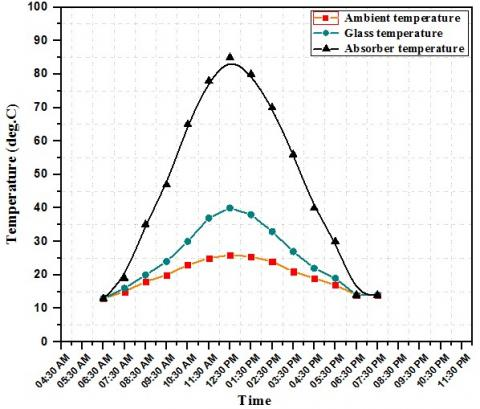Figure 4. Ambient, glass and absorber temperatures for SAH without heat storage materials at (4.47 kg/sec)

## 5.jpgFigure 5. Absorber temperature for three test caseswith4.47 kg/sec

The amount of heat that undergoes and rejected depending on the physical properties of used materials such as thermal conductivity, specific heat as shown in Table 2, it's seen especially on the PCM (paraffin wax) curve at the same interval time, the absorber temperature of the collector with PCM higher than collector with pure cement, because the thermal conductivity of paraffin wax is less than pure cement and this led to absorber temperature is high. In discharging time after 2:30 PM the storage heat materials start to reject heat to absorber, and the behavior of the curves is inverted (collector absorber with PCM less than pure cement), this led to paraffin wax keeping in heat for a longer time 2 hour compared with pure cement as shown in Figure 7.

## 6.jpgFigure 6. Output air temperature for three test cases with 4.47 g/sec

## 7.jpg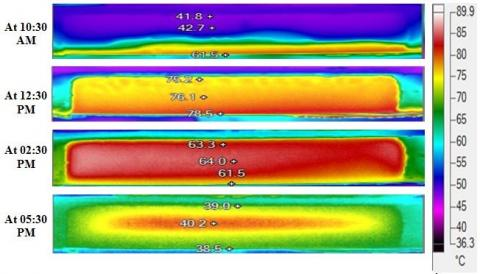Figure 7. Infrared camera picture for collector container filled with PCM different selected test time

## 8.jpgFigure 8. Collector useful heat gain for three test cases with 4.47 kg/sec

Figure 7 gives an infrared camera picture for collector container filled with PCM, melting behavior of paraffin wax in container cross-section are shown at different selected test time(charge and discharge), and absorber temperature as pointed on the picture, it is seen that at 5:30 PM the temperature still kept inside paraffin wax. The heat gains from the three collectors along the test time are shown in Figure 8.

Figure 9 shows the collector efficiency for the three cases, to show the efficiency clearly to make a comparison between them; it must take instantaneous efficiency for the three cases at the same selective time was taken in Figure 7 as shown in Figure 10, depending on absorber temperature for the three cases, output air temperature limit collector efficiency at each time as shown in equations 1 and 4, it seen that at charging time 10:30 AM and 12:30 PM paraffin wax gives higher collector efficiency than pure cement, but at discharge time 2:30 PM and 5:30 PM pure cement gives higher collector efficiency than paraffin wax as the same cause discussed in Figure 5 and 6. Figure 11 shows thermal storage efficiency depending on thermal calculations (useful heat energy and losses) from the solar air heater (collector) for the three test cases, it is seen that the collector thermal storage efficiency duration time was enhanced about 50% by using paraffin wax compared with pure cement, collectors give heat along 240 min, 120 min after 6:30 PM by using paraffin wax and pure cement respectively.

## 9.jpgFigure 9. Solar collector efficiency for three test cases along test days

## 10.jpg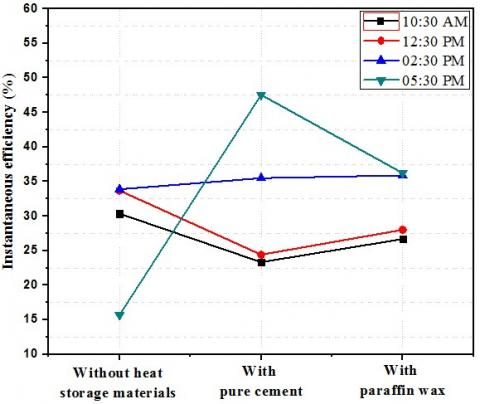Figure 10. Instantaneous efficiency of three collectors

## 11.jpgFigure 11. Thermal storage efficiency of three collectors

5. Conclusions

It can be concluded from this work that the latent storage heat material for paraffin wax as a PCM gives better thermal performance than sensible storage heat material in the solar air heater. The collector thermal storage efficiency duration time was enhanced by about 50% using paraffin wax compared with pure cement. The solar air heater gave heat along 240 min, 120 min after 6:30 PM by using paraffin wax and pure cement respectively.

Nomenclature
 A area (m2). G irradiation (W/m2) hc convective heat transfer coefficient (W/m2.k). hr the radiation heat transfer coefficient (W/m2 .k). hw the convective heat transfer coefficient by the wind (W/m2.k). K thermal conductivity(W/m.k). L distance (m). N number. Pr prandtl number. Q Energy. T temperature (oC). U heat loss coefficient (W/m2.k). V average velocity of the out let air(m/s) v air kinematic viscosity(m2/s) Greek symbols ε emittance η efficiency (%) ρ density(kg/m3) β collector tilt angle(deg) Subscripts a air. b collector bottom insulation b-a from the bottom to ambient. c collector. d duct e collector edge insulation e-a from the edge to ambient g gravitational constant (9.81 m2/s) g-a from the glass to ambient. p-g the absorber plate to glass cover. t top tb collector bottom insulation thickness (m). te collector edge insulation thickness (m).
References

 Enibe, S.O. (2002). Performance of a natural circulation solar air heating system with phase change material energy storage. Renewable Energy, 27(1): 69-86. https://doi.org/10.1016/S0960-1481(01)00173-2

 Enibe, S.O. (2003). Thermal analysis of a natural circulation solar air heater with phase change material energy storage. Renewable Energy, 28(14): 2269-2299. https://doi.org/10.1016/S0960-1481(03)00071-5

 Sharma, S.D., Iwata, T., Kitano, H., Sagara, K. (2005). Thermal performance of a solar cooker based on an evacuated tube solar collector with a PCM storage unit. Solar Energy, 78(3): 416-426. https://doi.org/10.1016/j.solener.2004.08.001.

 Alkilani, M.M., Sopian, K., Alghoul, M.A., Mat, S. (2009). Using a paraffin wax- aluminum compound as thermal storage material in solar air heater. ARPN Journal of Engineering and Applied Sciences, 4(10): 74-77.

 Saravanakumar, P.T., Mayilsamy, K. (2010). Forced convection flat plate solar air heaters with and without thermal storage. Journal of Scientific & Industrial Research, 69(12): 966-968. http://nopr.niscair.res.in/handle/123456789/10667

 Abdulmunem, R.A., Akram, H.A., Haqi, I.Q. (2016). Applicablity of using thermal storage materials in solar air heater. The Iraqi Journal for Mechanical and Material Engineering, 16(1): 76-86.

 Alkilani, M.M., Sopian, K., Mat, S. (2011). Fabrication and experimental investigation of PCM capsules integrated in solar air heater. American Journal of Environmental Sciences, 7(6): 542-546. https://doi.org/10.3844/ajessp.2011.542.546

 Jaffal, H.M. (2014). Theoritical analysis on thermal energy storage using phase change materials capsules for solar organic Rankine cycle power generation system. Nahrain University, College of Engineering Journal (NUCEJ), 17(l): 15-35. https://nahje.com/index.php/main/article/view/206

 Duffie, J.A., Beckman, W.A. (2013). Solar Engineering of Thermal Processes, 4th Edition. Copyright © 2013 John Wiley & Sons, Inc. https://doi.org/10.1002/9781118671603

 Abdulmunem, R.A., Jalil, M.J. (2018). Indoor investigation and numerical analysis of PV cells temperature regulation using coupled PCM/Fins. International Journal of Heat and Technology, 36(4): 1212-1222. https://doi.org/10.18280/ijht.360408

 Abdulmunem, R.A., Abdullateef, A.J., Hisham, A.H., Mohammed, H.J. (2018). Effect of colored filters on PV panels temperature and performance under Baghdad meteorological condition. Tikrit Journal of Engineering Sciences, 25(4): 45-49.

 Abdulmunem, R.A., Mohammed, H.J., Muhannad, Z.K. (2018). Modeling study of PV power plants in different Iraq’s governorates. The Iraqi Journal for Mechanical and Material Engineering, 18(4): 628-643.

 Hashim, A.H., Ali, H.N., Abdulmunem, R.A. (2017). An experimental investigation on the performance enhancement of photovoltaic/thermal panel using a tracking system and nanofluid (Al2O3). Engineering and Technology Journal, 35A(5): 493-508. https://www.iasj.net/iasj?func=article&aId=130209

 Hashim, A.H., Ali, H.N., Abdulmunem, R.A. (2015). Indoor investigation for improving the hybrid photovoltaic/thermal system performance using nanofluid (Al2O3-Water). Engineering & Technology Journal, 33A(4): 889-901.

 Hashim, A.H., Ali, H.N., Abdulmunem, R.A. (2015). Improving of the photovoltaic/thermal system performance using water cooling technique. IOP Conf. Series: Materials Science and Engineering, 78(012020): 1-9. https://doi.org/10.1088/1757-899X/78/1/012020

 Hussien, H., Hasanuzzaman, M., Numan, A.H., Abdulmunem, R.A. (2014). Enhance photovoltaic/thermal system performance by using nanofluid. 3rd IET International Conference on Clean Energy and Technology (CEAT). https://doi.org/10.1049/cp.2014.1515